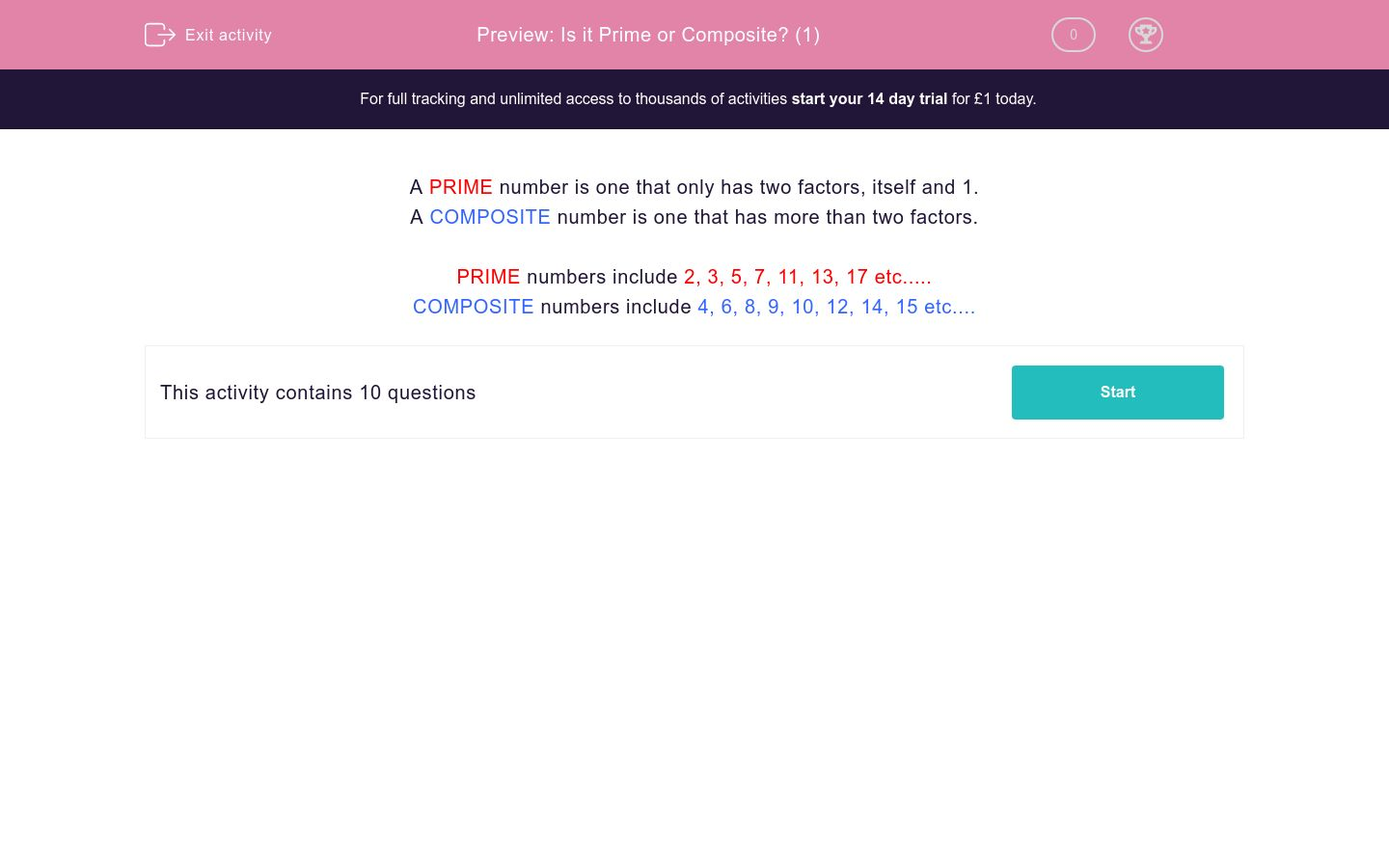# Is it Prime or Composite? (1)

In this worksheet, students decide whether or not a given number is prime or composite.Key stage:  KS 2

Curriculum topic:   Maths and Numerical Reasoning

Curriculum subtopic:   Special Numbers

Difficulty level:### QUESTION 1 of 10

A PRIME number is one that only has two factors, itself and 1.

A COMPOSITE number is one that has more than two factors.

PRIME numbers include 2, 3, 5, 7, 11, 13, 17 etc.....

COMPOSITE numbers include 4, 6, 8, 9, 10, 12, 14, 15 etc....

Is the following number prime or composite?

12

Prime

Composite

Is the following number prime or composite?

11

Prime

Composite

Is the following number prime or composite?

17

Prime

Composite

Is the following number prime or composite?

27

Prime

Composite

Is the following number prime or composite?

37

Prime

Composite

Is the following number prime or composite?

57

Prime

Composite

Is the following number prime or composite?

49

Prime

Composite

Is the following number prime or composite?

59

Prime

Composite

Is the following number prime or composite?

2

Prime

Composite

Is the following number prime or composite?

51

Prime

Composite

• Question 1

Is the following number prime or composite?

12

Composite
EDDIE SAYS
12 can also be divided by 2, 3, 4 and 6
• Question 2

Is the following number prime or composite?

11

Prime
EDDIE SAYS
11 can only be divided by itself and 1
• Question 3

Is the following number prime or composite?

17

Prime
EDDIE SAYS
17 can only be divided by itself and 1
• Question 4

Is the following number prime or composite?

27

Composite
EDDIE SAYS
27 can also be divided by 3 and 9
• Question 5

Is the following number prime or composite?

37

Prime
EDDIE SAYS
37 can only be divided by itself and 1
• Question 6

Is the following number prime or composite?

57

Composite
EDDIE SAYS
57 can also be divided by 3 and 19
• Question 7

Is the following number prime or composite?

49

Composite
EDDIE SAYS
49 can also be divided by 7
• Question 8

Is the following number prime or composite?

59

Prime
EDDIE SAYS
59 can only be divided by itself and 1
• Question 9

Is the following number prime or composite?

2

Prime
EDDIE SAYS
2 can only be divided by itself and 1
• Question 10

Is the following number prime or composite?

51

Composite
EDDIE SAYS
51 can also be divided by 3 and 17
---- OR ----

Sign up for a £1 trial so you can track and measure your child's progress on this activity.

### What is EdPlace?

We're your National Curriculum aligned online education content provider helping each child succeed in English, maths and science from year 1 to GCSE. With an EdPlace account you’ll be able to track and measure progress, helping each child achieve their best. We build confidence and attainment by personalising each child’s learning at a level that suits them.

Get started